# Chords of a circle

Chords of a circle
Go back to  'Circles'

Grandfather: What are you searching for on the Internet?Sam: My teacher has asked me to find a real-life example of a chord.
Grandfather: That's very simple! A real-life example of a chord and an arc is a bow.
Sam: I hadn't thought of that! Thank you, Grandfather!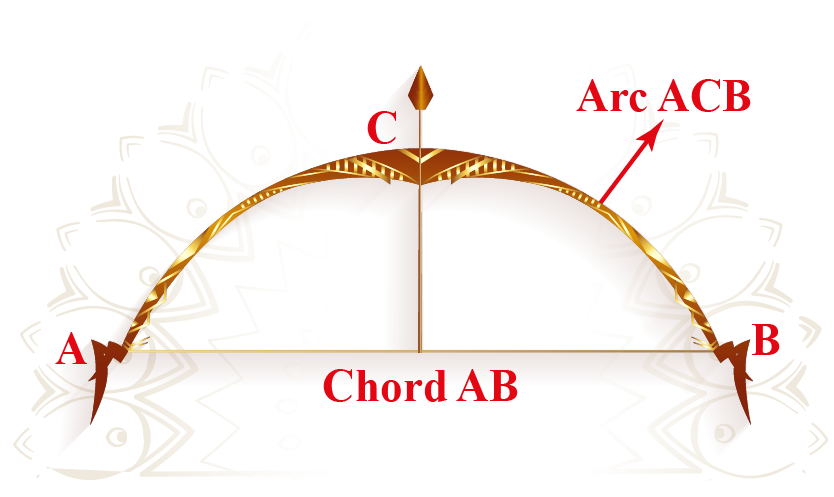Would you like to learn more about the chords of a circle?

Come, let us learn in detail about the chords of a circle theorems, intersecting chords theorem, congruent circles, and diameter in this mini-lesson.

## Lesson Plan

 1 What Are Chords in a Circle? 2 Important Notes on Chords of a Circle 3 Solved Examples of Chords of a Circle 4 Challenging Questions on Chords of a Circle 5 Interactive Questions on Chords of a Circle

## What Are Chords in a Circle?

A chord of a circle is any line segment joining two points on the circumference of the circle.

## What Are Equal Chords in a Circle?

Chords that are equidistant from the center of a circle are considered equal chords.

Now, we will discuss some other fundamental properties related to circles.

### Equal Chords Theorem

Theorem: Equal chords of a circle are equidistant from the center.

The following figure shows two equidistant chords $$AB$$ and $$CD$$ of a circle with center $$O$$

The distances of the two chords are $$OX$$ and $$OY$$ respectively.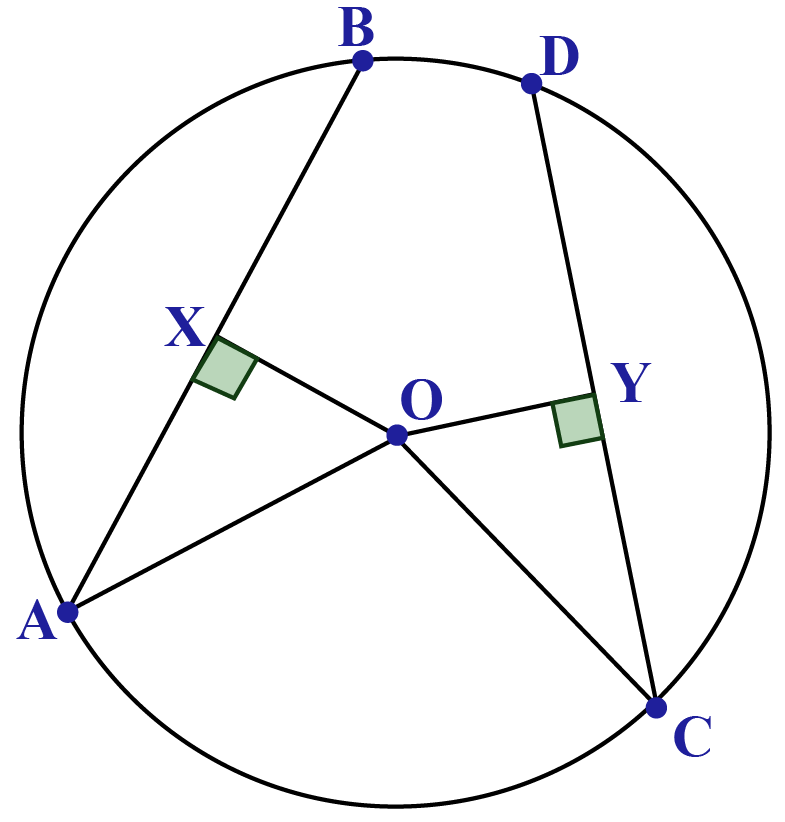We need to show that $$OX$$ = $$OY$$.

We have joined $$OA$$ and $$OC$$.

Note that since $$OX$$ is perpendicular to $$AB$$, it must also bisect $$AB$$.

Similarly, since $$OY$$ is perpendicular to $$CD$$, it must also bisect $$CD$$.

Since $$AB$$ = $$CD$$, we have

$\raise.5ex\hbox{1}\kern-.1em/\kern-.15em\lower.25ex\hbox{2} {\rm{ }}\left( {AB} \right){\rm{ }} = {\rm{ }}AX{\rm{ }} = {\rm{ }}\raise.5ex\hbox{1}\kern-.1em/\kern-.15em\lower.25ex\hbox{2} {\rm{ }}\left( {CD} \right){\rm{ }} = {\rm{ }}CY$

Proof: Compare $$\Delta\mathrm O\mathrm A\mathrm X$$ with $$\Delta\mathrm O\mathrm C\mathrm Y$$.

1. $$OA$$ = $$OC$$ (radii of the same circle)

2.$$AX$$ = $$CY$$ (we just proved this above)

3. $$\angle {\rm{OXA }} = \angle {\rm{OYC }} = {\rm{ }}{90^0}$$

By the RHS criterion, $$\Delta {\rm{OAX}} \cong \Delta {\rm{OCY}}$$.

Thus, $$OX$$ = $$OY$$, which means that $$AB$$ and $$CD$$ are equidistant from $$O$$.

The converse theorem also holds.

Converse: Two chords of a circle which is equidistant from its center must have the same length.

Proof: Referring to the previous figure, we compare $$\Delta\mathrm{OAX}$$ with $$\Delta\mathrm{OCY}$$

1. $$OA$$ = $$OC$$ (radii of the same circle)

2. $$OX$$ = $$OY$$ (given)

3. $$\angle {\rm{OXA }} = \angle {\rm{OYC }} = {\rm{ }}{90^0}$$

By the RHS criterion again, the two triangles are congruent, and hence $$AX$$ = $$CY$$. Since $$OX$$ and $$OY$$ must also bisect $$AB$$ and $$CD$$ respectively (why?), we have

${\rm{AB }} = {\rm{ }}2{\rm{AX }} = {\rm{ }}2{\rm{CY }} = {\rm{ CD}}$

Thus, the two chords are of equal length.

## Length of Chord Calculator

Set Radius and Length of perpendicular, the calculator will calculate the length of the chord.

## What Are Considered as Unequal Chords in a Circle?

Chords that are not equidistant from the center of a circle are considered unequal chords.

### Unequal Chords Theorem

Theorem: For two unequal chords of a circle, the larger chord will be closer to the center than the smaller chord.

This should be obvious when you observe the figure shown below.

As your chord moves closer to the center, it increases in length.

The diameter is the largest possible chord in any circle.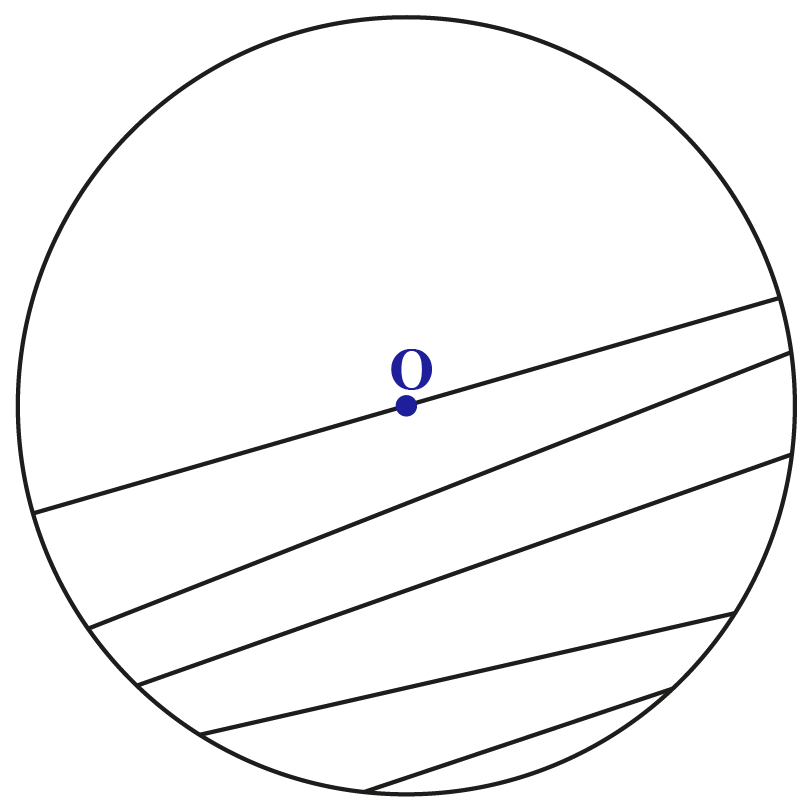The following figure shows two chords $$AB$$ and $$CD$$ of a circle with center $$O$$, such that $$AB$$ > $$CD$$.

OX and OY are perpendiculars to the two chords from the center; this means that they will bisect the two chords $$AB$$ and $$CD$$ respectively.

We need to prove that $$OX$$ < $$OY$$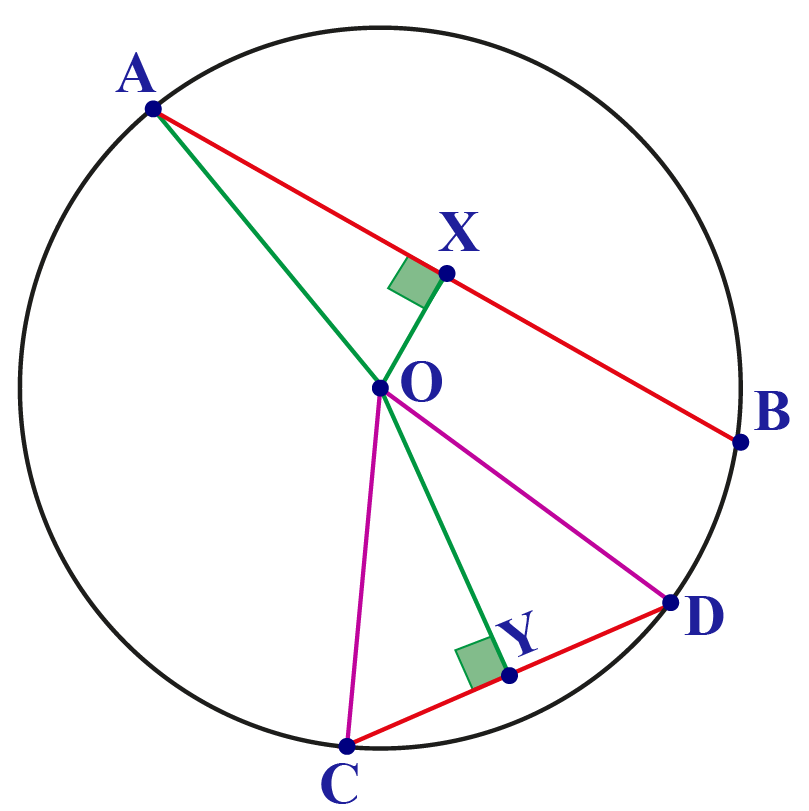Join $$OA$$ and $$OC$$.

Proof: The proof involves a simple application of the Pythagoras theorem.

We note that in $$\Delta\mathrm O\mathrm A\mathrm X$$,

${\rm{O}}{{\rm{X}}^{\rm{2}}} + {\rm{ X}}{{\rm{A}}^{\rm{2}}} = {\rm{ O}}{{\rm{A}}^{\rm{2}}}$

Similarly, in ∆OCY,

${\rm{O}}{{\rm{Y}}^{\rm{2}}}{\rm{ + Y}}{{\rm{C}}^{\rm{2}}}{\rm{ = O}}{{\rm{C}}^{\rm{2}}}$

Since $$OA$$ = $$OC$$ (radii of the circle), we have

${\rm{O}}{{\rm{X}}^{\rm{2}}}{\rm{ + X}}{{\rm{A}}^{\rm{2}}}{\rm{ = O}}{{\rm{Y}}^{\rm{2}}}{\rm{ + Y}}{{\rm{C}}^{\rm{2}}}$

Both the sides above have a sum of two terms.

If a term on one side is greater than the corresponding term on the other, then the other term on the first side must be less than the corresponding term on the second side.

That is, since $$XA$$ > $$YC$$, this must mean that

${\rm{OX < OY}}$

Thus, $$AB$$ is nearer to the center than $$CD$$.

## Is Diameter a Chord?

Yes, the diameter is a chord, since it also joins two points lying on the circumference of a circle.

A chord that passes through the center of the circle is known as the diameter chord, or simply a diameter.

In the following figure, AB and CD are two diameter chords of the circle.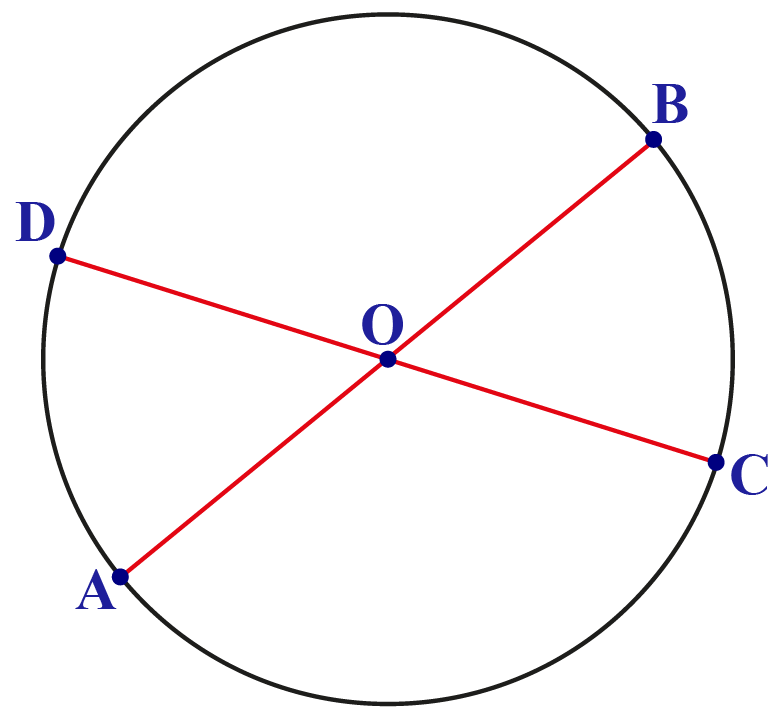Important Notes
• A line is drawn from the center of a circle to bisect a chord is perpendicular to the chord.
• The perpendicular from the center to the chord bisects the chord.
• While comparing the length of two arcs, the length of the chord belongs to greater arc length is greater.

## Solved Examples

 Example 1

In the given circle, O is the center, and chord AB is 16 cm.

Find the length of AD if OM is the radius of the circle.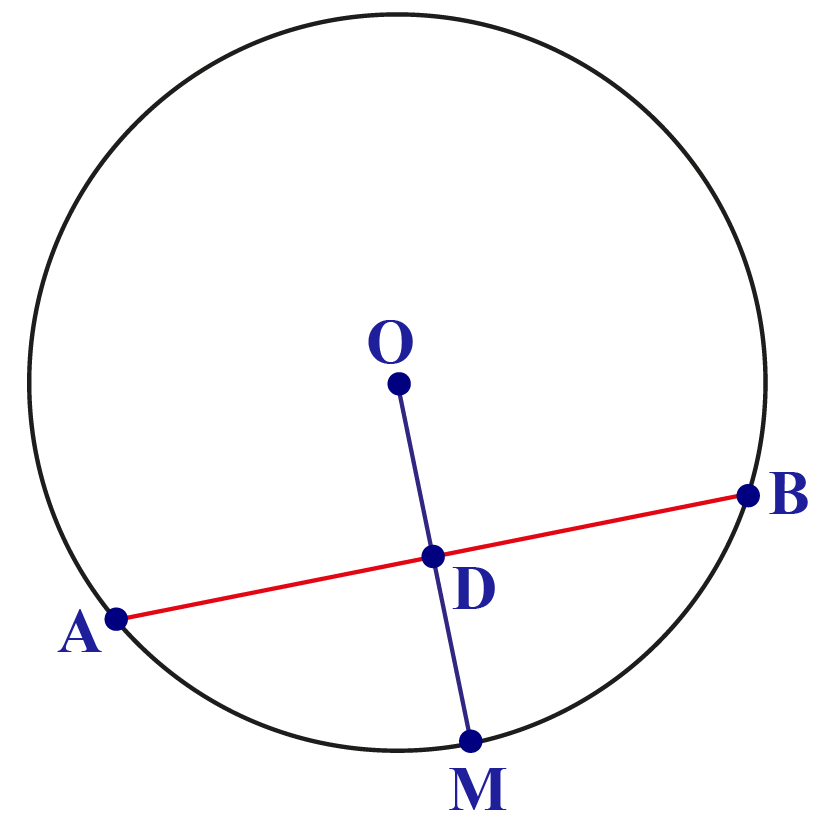Solution

We know that the radius of a circle is always perpendicular to the chord and acts as a perpendicular bisector.

Therefore,
\begin{align}\text{AD}&= \frac{1}{2} \times \text{AB} \\&= \frac{16}{2} \\&= 8\end{align}

 Hence, AD = 8 cm.
 Example 2

In the given circle, O is the center with a radius of 5 cm.

Find the length of chord AB if the length of the perpendicular drawn from the center is 4 cm.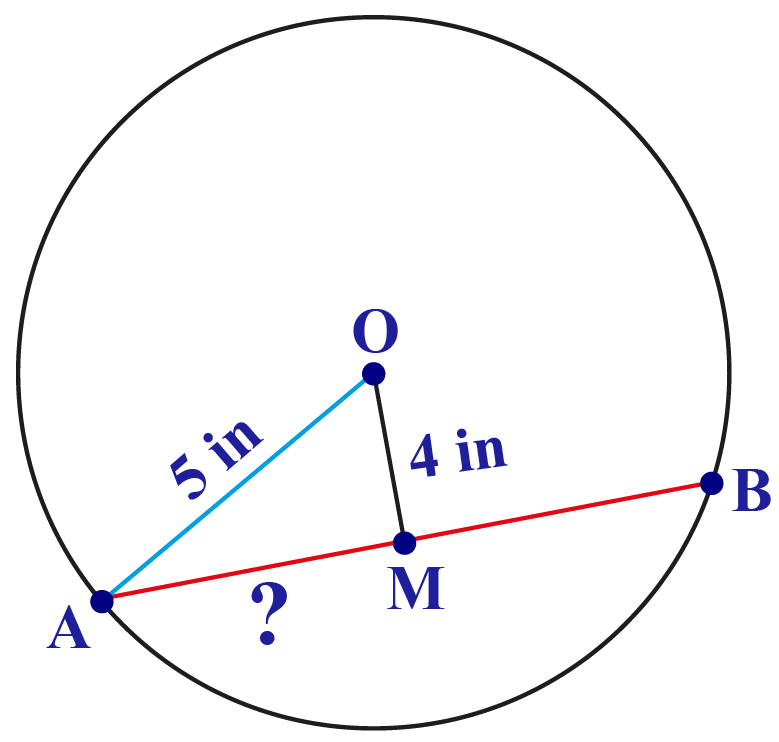Solution

Since OM is perpendicular to AB, △AOM will be a right-angled triangle.

In △AOM, from Pythagoras theorem
${OA^2} = {OM^2} + {AM^2}$
After transposition  of terms, we get
${AM^2} = {OA^2} - {OM^2}$
On substituting the values, $A{M^2} = {5^2} - {4^2}$
\begin{align}\text{AM}&=\sqrt{9} \\&= 3\end{align}
Therefore,\begin{align}\text{Chord AB}&= 2 \times 3 \\&= 6\end{align}

 $$\therefore$$ Chord AB = 6 cmChallenging Questions

A circle has a radius of 25 inches. Find the distance between the two parallel chords which are 48 inches and 40 inches in length if they lie on

• opposite sides of the center.
• same side of the center.

## Interactive Questions

Here are a few activities for you to practice.

Select/Type your answer and click the "Check Answer" button to see the result.

## Let's Summarize

The mini-lesson targeted the interesting concept of chords of a circle. We hope you enjoyed learning about the diameter and chords of the circle theorem with the simulation and practice questions. Now, equal chords in a circle and unequal chords in a circle is no more difficult for you.

## About Cuemath

At Cuemath, our team of math experts is dedicated to making learning fun for our favorite readers, the students!
Through an interactive and engaging learning-teaching-learning approach, the teachers explore all angles of a topic.
Be it worksheets, online classes, doubt sessions, or any other form of relation, it’s the logical thinking and smart learning approach that we, at Cuemath, believe in.

## 1. How do you prove two chords are equal?

If two chords are equidistant from the center of a circle, then the length of the chords are equal.

## 2. What happens when two chords intersect in a circle?

When two chords intersect each other, the product of their segments is equal.

## 3. Is radius a chord?

Radius is not a chord since it does not join two points lying on the circumference of a circle.

## 4. How do you find the radius of a circle with chord length?

By substituting half of the chord length and the length of the perpendicular in Pythagoras theorem, one can find the radius.

## 5. How many chords are in a circle?

A circle can have infinite numbers of chord.

## 6. What is the largest chord of a circle?

Diameter is the largest chord of the circle.

More Important Topics
Numbers
Algebra
Geometry
Measurement
Money
Data
Trigonometry
Calculus